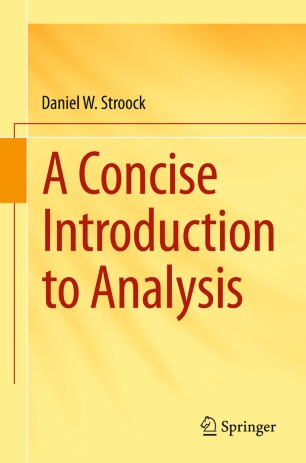# A Concise Introduction to Analysis

• Daniel W. StroockTextbook

1. Front Matter
Pages i-xii
2. Daniel W. Stroock
Pages 1-41
3. Daniel W. Stroock
Pages 43-58
4. Daniel W. Stroock
Pages 59-97
5. Daniel W. Stroock
Pages 99-126
6. Daniel W. Stroock
Pages 127-173
7. Daniel W. Stroock
Pages 175-208
8. Back Matter
Pages 209-218

### Introduction

This book provides an introduction to the basic ideas and tools used in mathematical analysis. It is a hybrid cross between an advanced calculus and a more advanced analysis text and covers topics in both real and complex variables. Considerable space is given to developing Riemann integration theory in higher dimensions, including a rigorous treatment of Fubini's theorem, polar coordinates and the divergence theorem. These are used in the final chapter to derive Cauchy's formula, which is then applied to prove some of the basic properties of analytic functions.

Among the unusual features of this book is the treatment of analytic function theory as an application of ideas and results in real analysis. For instance, Cauchy's integral formula for analytic functions is derived as an application of the divergence theorem. The last section of each chapter is devoted to exercises that should be viewed as an integral part of the text.

A Concise Introduction to Analysis should appeal to upper level undergraduate mathematics students, graduate students in fields where mathematics is used, as well as to those wishing to supplement their mathematical education on their own. Wherever possible, an attempt has been made to give interesting examples that demonstrate how the ideas are used and why it is important to have a rigorous grasp of them.

### Keywords

Mathematical Analysis Advanced Calculus Riemann Integration Theory Fubini's Theorem Polar Coordinates Divergence Theorem Cauchy's Formula Analytic Function Theory Real Analysis Cauchy's Integral Formula Analytic Functions Divergence Theorem

#### Authors and affiliations

• Daniel W. Stroock
• 1
1. 1.Department of MathematicsMassachusetts Institute of TechnologyCambridgeUSA